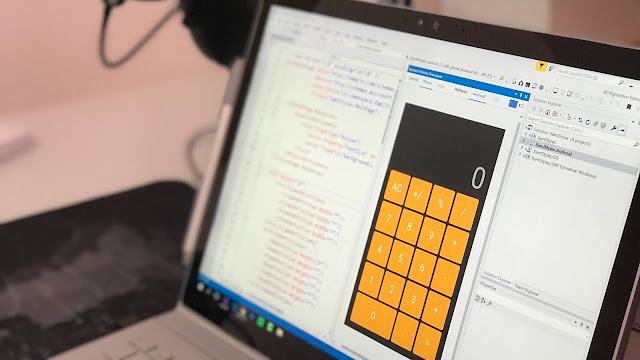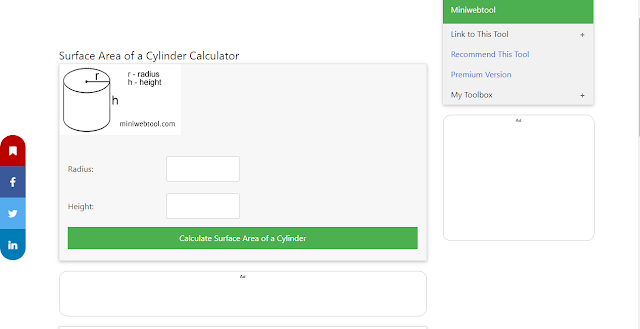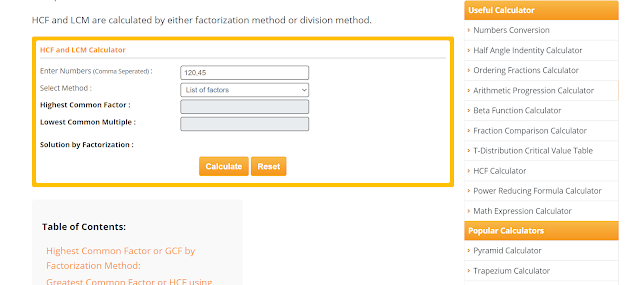# 3 Common Online Calculators Recommended For High-School Students 😍🔥

It is important to understand why high school students need to use online calculators before we dig into the categories they opt for. When you are acquiring education at the high school level, everything is not spoon-fed. Students are expected to attend lectures, prepare for tests and work on homework assignments. In an overall manner, they have to complete several tasks in a limited daily span. Some courses involve thorough calculations for which it is advisable to use online calculators.

Physics and mathematics are the two disciplines for which students have to use calculators. For instance, if you have to solve fractions, the best option is to select a good website and use a quality online tool. Students use Mera Calculator and countless other websites to solve mathematics problems and numerical questions.This website has easy to use calculation applications that can be used without tolerating any cost. Along with that, it has a reputation and students do not have to worry about correctness problems. Apart from fractions, high school students are taught various other mathematics topics including HCF (Highest Common Factor) and LCM (Lowest Common Multiple).

## Context

• Common Online Calculators For High-School Students
• Fraction calculator
• Cylinder Calculator
• HCF calculator
• Conclusion

## Common Online Calculators For High-School Students

### 1. Fraction calculatorOne of the few subjects that involve calculations at the high school level is Mathematics. A number of topics are taught to students and fractions is one of them. It is not easy to strengthen concepts if you are studying it for the first time. In order to improve the clarity level, students are told to practice regularly and solve as many problems as possible. This helps in developing a strong command.  Let us have a look at the points given below to see how you can use it.

This calculator basically converts the decimal value to fractions. In other words, you have to enter the decimal value as input and its equivalent fraction value will be shown. For instance, suppose that you have the number 0.45. To convert it to fraction, enter this value in the input box. After that, you would see “45/100” as the fraction value.

At the high school, this topic is taught on a major scale because the concepts are further used for professional education. For instance, if you are acquiring a professional degree in mechanics or mathematics, it would be impossible to get good scores if you don’t know fractions inside out. In order to practice properly and solve the maximum count of questions, this tool will be helpful.

### 2. Cylinder CalculatorAt high school, different sub-disciplines of mathematics are taught to students. One of them is geometry. Students have to calculate the area of different geometrical shapes. Some of them are quite easy and you can determine the answers on fingertips. For instance, if you have to calculate the area of a square, it can be done orally if the values are not tough. However, if you have to determine the volume or total surface area of a cylinder, a calculator is definitely needed.

This calculator is quite simple to use and only two input values have to be entered by the user. Once you punch in these two values, the total surface area and volume can be found out. Let us check an example to see how this works.

#### Example

Suppose that you wish to calculate the total surface area and volume of a cylinder. The value of radius is 10 and the height is 14. When you enter the values, the following outputs will be shown to you.

• Total Surface area = 1507
• Volume = 879

It is quite hard to perform these calculations without using online calculators. For instance, the formula of the total surface area is 2πr(h+r). Even if you have good mathematics skills, learning this formula and using it correctly will not be an easy goal to achieve.

### 3. HCF CalculatorHCF (Highest Common Factor) is a topic related to algebra. This calculator comes in handy if you don’t want to spend time on breaking down each number and finding the multiples. It helps in getting quick accurate answers when you do not have a lot of time available. For instance, at times, students have to complete a mathematics assignment in limited time. They cannot afford to perform detailed steps as this creates delays. The purpose of an HCF calculation tool is solving a large count of questions without consuming a lot of time.

At times, using online tools becomes more of a disadvantage when the interface is hard to interpret. There is nothing complex about this tool because all the user has to do is enter the two input numbers. For instance, if the HCF of 10 and 16 has to be determined, enter them in the required text boxes.  In this case, the value of HCF will be 2. The overall process will not require more than 30 seconds. From the student’s perspective, this is a major plus point.

## Conclusion

When you are performing calculations, the eventual goal is getting correct answers.  The number of steps you perform or the time consumed are not important factors. It is always a good option to use online calculators whether you are solving problems related to mathematics, physics or any other subject. Some students have the opinion that getting basic information about the formula is sufficient for solving problems manually. This is not correct. Knowing the formula and applying it without making mistakes are two different things. If you have time-related pressures, errors can be made even if you know the calculation process.

There is no reason for not using these online soft wares because they come for free. If you are using a fraction calculator, there is no reason to spend any money even if you are solving 100 problems. Most online calculators are very easy to use. Hence, not having a lot of technical knowledge does not make any difference. By entering values, you can get correct results without going through any hassle.